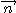### Solution : Field on the axis of a circular opening of a plane - corrected exercises Gauss theorem

The Load distribution can be seen as the result of the superposition of an infinite plane of a surface density of charge s and a disc with a density - s.
The field at a point M of the axis  according to the principle of superposition is the vector sum of the fields created by each of these distributions taken separately.
Either:
Notingthe unit vector normal to the plane and oriented to the point M, z the coast of the point M from the center of the circular opening ,we have , using conventional demonstrated results in progress (demonstrate need to know!):
Finally :